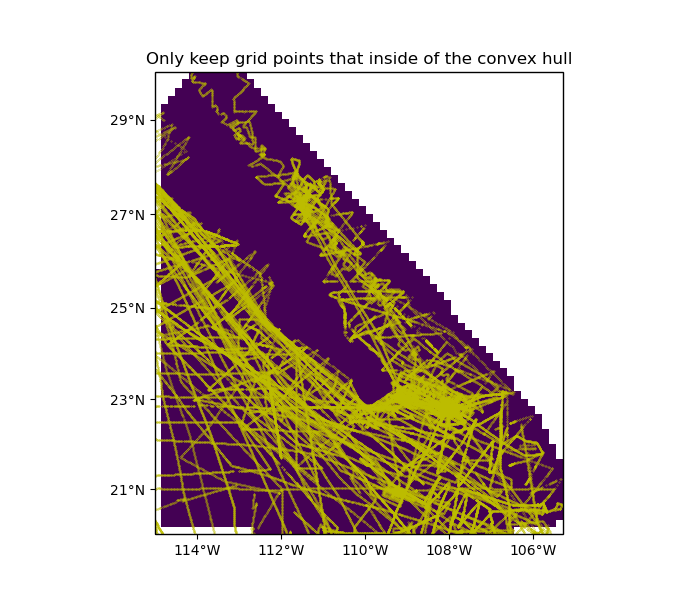# Mask grid points by convex hull¶

Sometimes, data points are unevenly distributed. In such cases, we might not want to have interpolated grid points that are too far from any data point. Function verde.convexhull_mask allows us to set grid points that fall outside of the convex hull of the data points to NaN or some other value.Out:

[[False False False ... False False False]
[False  True  True ...  True False False]
[False  True  True ...  True  True False]
...
[False False False ... False False False]
[False False False ... False False False]
[False False False ... False False False]]
/home/travis/miniconda/envs/testing/lib/python3.6/site-packages/cartopy/mpl/geoaxes.py:782: MatplotlibDeprecationWarning: Passing the minor parameter of set_xticks() positionally is deprecated since Matplotlib 3.2; the parameter will become keyword-only two minor releases later.
return super(GeoAxes, self).set_xticks(xticks, minor)
/home/travis/miniconda/envs/testing/lib/python3.6/site-packages/cartopy/mpl/geoaxes.py:829: MatplotlibDeprecationWarning: Passing the minor parameter of set_yticks() positionally is deprecated since Matplotlib 3.2; the parameter will become keyword-only two minor releases later.
return super(GeoAxes, self).set_yticks(yticks, minor)
/home/travis/build/fatiando/verde/examples/convex_hull_mask.py:50: UserWarning: Tight layout not applied. The left and right margins cannot be made large enough to accommodate all axes decorations.
plt.tight_layout()


import matplotlib.pyplot as plt
import cartopy.crs as ccrs
import pyproj
import numpy as np
import verde as vd

# The Baja California bathymetry dataset has big gaps on land. We want to mask
# these gaps on a dummy grid that we'll generate over the region just to show
# what that looks like.
data = vd.datasets.fetch_baja_bathymetry()
region = vd.get_region((data.longitude, data.latitude))

# Generate the coordinates for a regular grid mask
spacing = 10 / 60
coordinates = vd.grid_coordinates(region, spacing=spacing)

# Generate a mask for points. The mask is True for points that are within the
# convex hull. We can provide a projection function to convert the coordinates
# before the convex hull is calculated (Mercator in this case).
data_coordinates=(data.longitude, data.latitude),
coordinates=coordinates,
projection=pyproj.Proj(proj="merc", lat_ts=data.latitude.mean()),
)

# Create a dummy grid with ones that we can mask to show the results. Turn
# points that are outside of the convex hull into NaNs so they won't show up in
# our plot.
dummy_data = np.ones_like(coordinates)

# Make a plot of the masked data and the data locations.
crs = ccrs.PlateCarree()
plt.figure(figsize=(7, 6))
ax = plt.axes(projection=ccrs.Mercator())
ax.set_title("Only keep grid points that inside of the convex hull")
ax.plot(data.longitude, data.latitude, ".y", markersize=0.5, transform=crs)
ax.pcolormesh(*coordinates, dummy_data, transform=crs)
vd.datasets.setup_baja_bathymetry_map(ax, land=None)
plt.tight_layout()
plt.show()


Total running time of the script: ( 0 minutes 2.185 seconds)

Gallery generated by Sphinx-Gallery